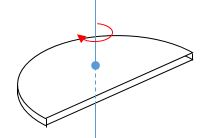# SemicircleThe moment of inertia of a uniform, semi-circular disc relative to the perpendicular axis through its center of mass is $I = \gamma m r^2$, where $m$ is the mass and $r$ the radius of the semi-circular disc. Determine the constant $\gamma$.

×